Constructing a copy of a given angle

Practical Geometry
Concept wise

Suppose we are given angle A

We need to draw its copy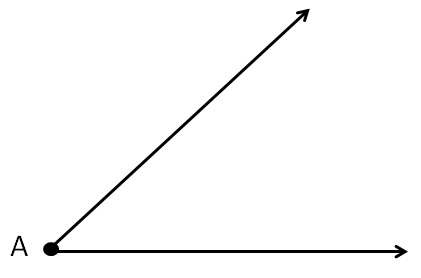1.Draw a ray OP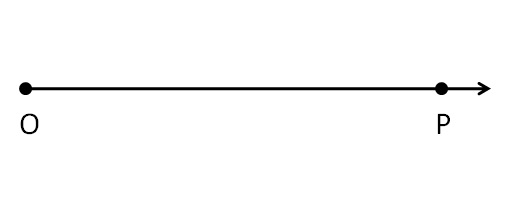1. From point A, draw an arc of any radius
intersecting the sides at B & C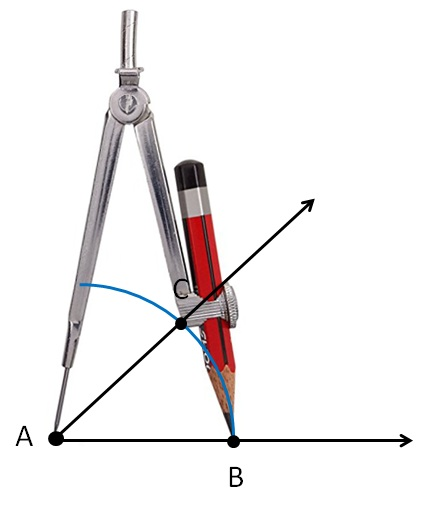2. Using compass opened the same length,

draw an arc with point O as the center,

cutting OP at Q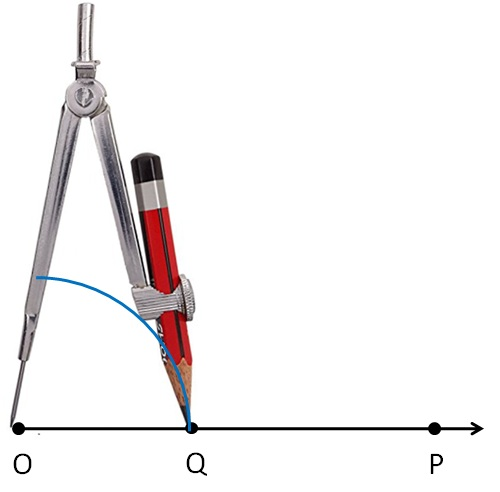3. Now, back to our angle A.
Open compass to length BC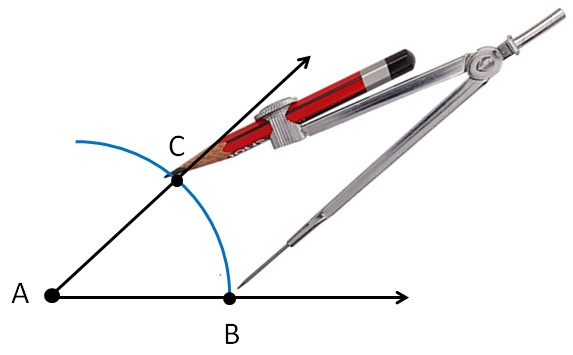Put pointed end of compass on point Q, and draw an arc.
The point where it cuts previous arc is point R.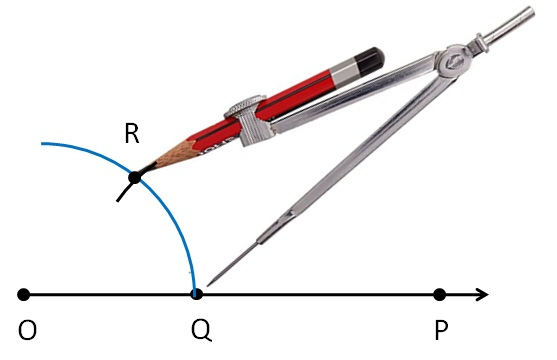5. Join OR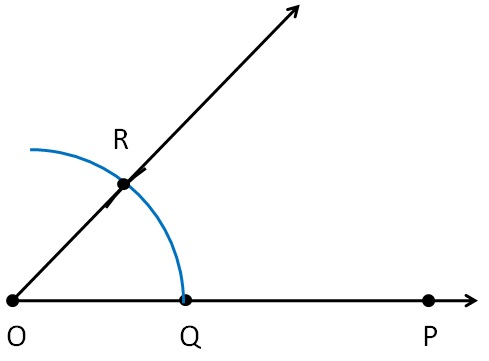Thus, ∠ ROQ is the copy of ∠ A

Learn in your speed, with individual attention - Teachoo Maths 1-on-1 Class